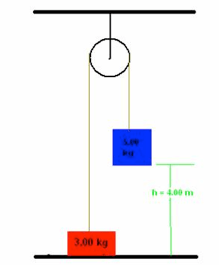Power is the rate at which work is done. Any rate is that quantity measured over a period of time, so power is work divided by time.

Power is measured in watts or kilowatts (a watt is a relatively small unit).

## Machines

Why do we have so many machines and what do they do for us?

All the machines in the world (and just think of how many you have around your house) are composed of just 6 simple machines.

A simple machine is something that does work with only one movement. A machine is something that makes work easier. Technically, a machine is a device that multiplies force at the expense of distance, or multiplies distance at the expense of force. When you put 2+ simple machines together, you have a compound machine. A compound machine is not necessarily complicated! Your pencil is a compound machine (point is a wedge, pencil is a lever)!

Try the following:

1) Water flows over a section of Niagara Falls at the rate of 1.2 X 1kg/s and falls 50 m. How much power is generated by the falling water?

Answer: (5.9 X 10^8W)

2) A ski jumper starts from rest 50.0 m above the ground on a frictionless track, and flies off the track at an angle of 45.0° above the horizontal and at a height of 10.0 m above the level ground. Neglect air resistance.

A. What is his speed when he leaves the track? (Answer: 28 m/s)

B. Where does he land relative to the end of the track? (Answer: 89.0 m beyond end of track)

3) Two objects are connected by a light string passing over a light, frictionless pulley as in the figure below. The 5.00 kg object is released from rest at a point 4.00 m above the floor.

A. Determine the speed of each object when the 2 pass each other.(Answer: 3.15 m/s)

B. Determine the speed of each object at the moment the 5.00 kg objects hits the floor.(Answer: 4.43 m/s)

C. How much higher does the 3.00 kg object travel after the 5.00 kg object hits the floor?(Answer: 1 m)(source)